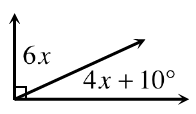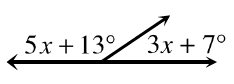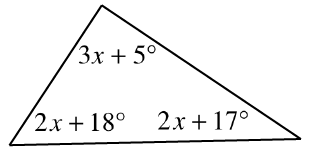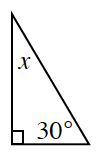### Home > CCG > Chapter 2 > Lesson 2.2.2 > Problem2-76

2-76.

For each diagram below, solve for $x$. Explain what relationship(s) from your Angle Relationships Toolkit you used for each problem.

1.What is the sum of the measures of the two angles in degrees?

1.What is the sum of the measures of the two angles in degrees?

1.What is the sum of the angles in a triangle?

1.How many degrees is the right angle?